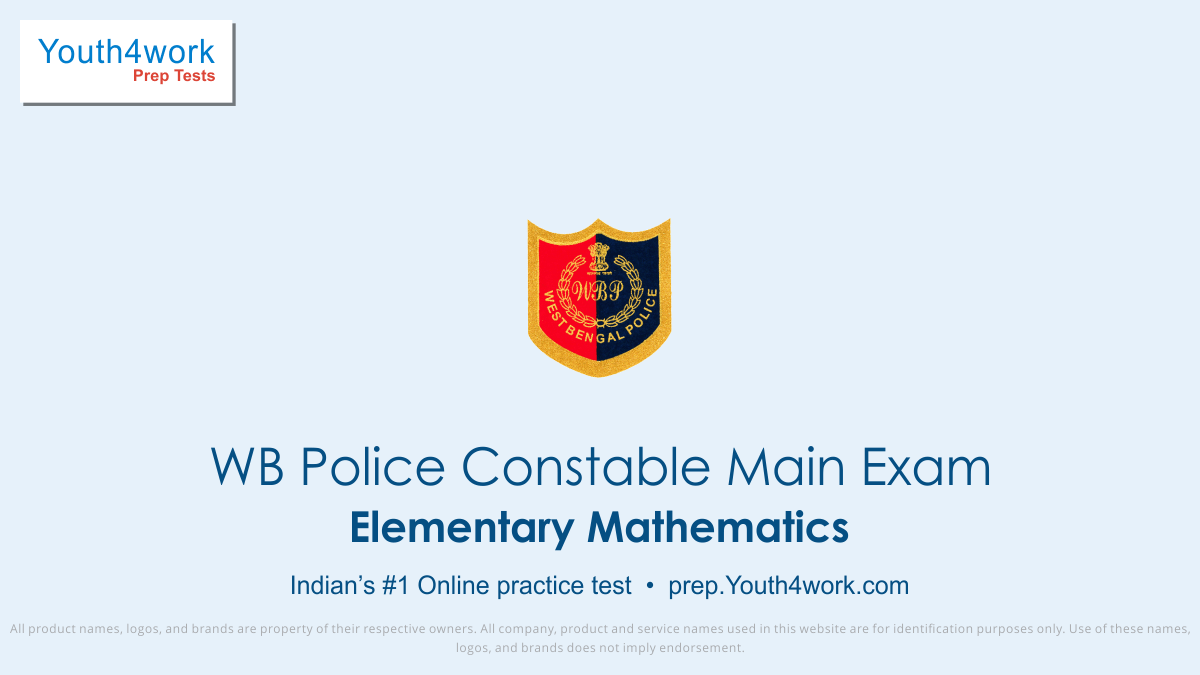# Examen online de práctica para Elementary Mathematics WB Police Constable Main Exam

Mixture and Alligation Mixture and Alligation

Time and Work Time and Work

Compound Interest Compound Interest

Simple Interest Simple Interest

Probability in mathematics depends mainly on two factors, events and outcomes. Probability is the likelihood of one or more events happening divided by the number of possible outcomes, for better understanding of this concept take this Online Mock Test and practice as many probability math problems as possible.

Click on this link and practice Youth4work Practice Papers and Online Mock Test Series for Quantitative Aptitude and score well. Frequently asked Average MCQs in WB Police Constable Exam are included in this Online Mock Test here.

Practice ratio and proportion math problems for alloys, mixtures and different kinds of arrangement in this free Online Mock Test which has been updated as per the pattern of questions asked in WBP Constable Exam.

Simplifying makes an algebraic expression easily understandable and solvable, Simplification math problems can be a high scoring area in the Mathematics section of the approaching WB Police Constable Prelims Exam.